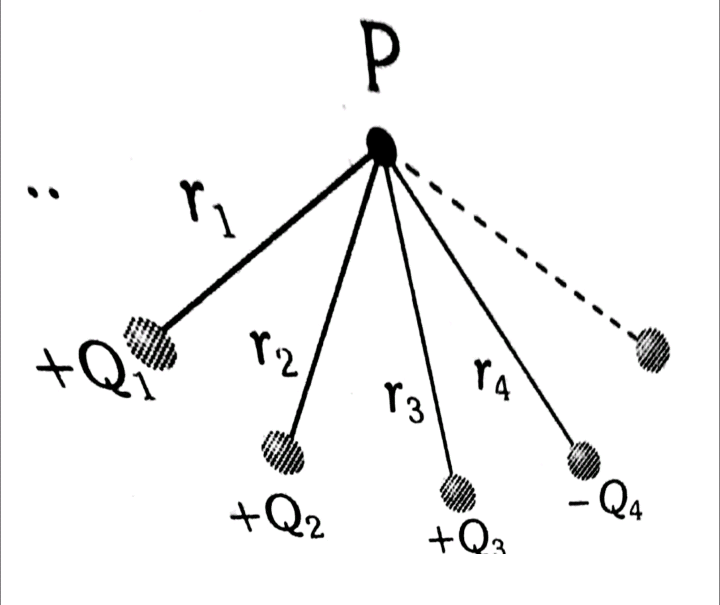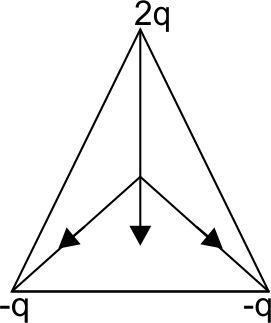# What will be the value of E and V at the centroid of equilateral if we place charges 2q, –q and -q at the vertices of the equilateral triangle? Option 1) E is not equal to 0 and V is equal to zero Option 2) V is not equal to 0 and E is equal to zero Option 3) Both are equal to zero Option 4) None of above

As we learnt in

Superposition of Electric field -

The resultant electric field at any point is equal to the vector sum of all the electric fields.

- wherein

and

Potential of a System of Charge -

- whereinElectric field of all the charges do not cancel each other as shown in the figure.Potential of positive charge is neutralised by other negative charge.

Hence  net potential = 0

Option 1)

E is not equal to 0 and V is equal to zero

This option is correct

Option 2)

V is not equal to 0 and E is equal to zero

This option is incorrect

Option 3)

Both are equal to zero

This option is incorrect

Option 4)

None of above

This option is incorrect

### Preparation Products

##### Knockout BITSAT 2021

It is an exhaustive preparation module made exclusively for cracking BITSAT..

₹ 4999/- ₹ 2999/-